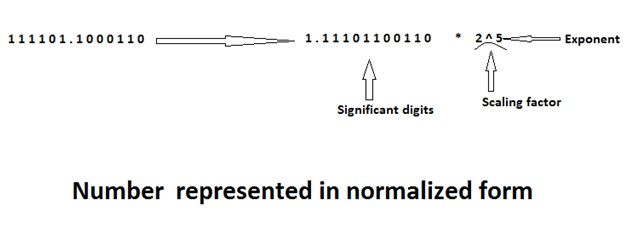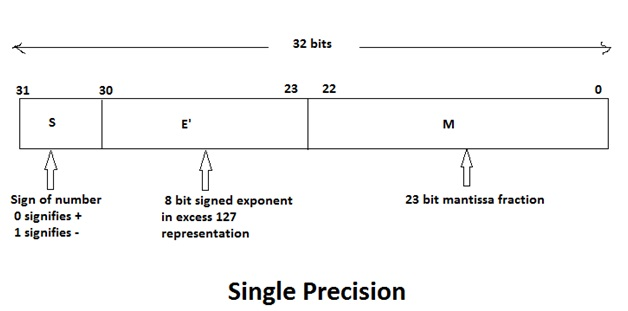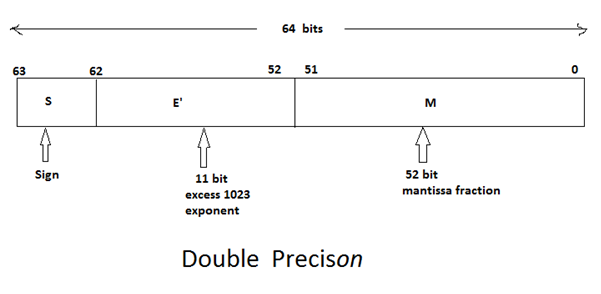# Floating point representation | Computer Science Organization

In this article, we will learn about the floating point representation and IEEE Standards for floating point numbers.
Submitted by Shivangi Jain, on August 21, 2018

## Floating point representation

• In floating point representation, the computer must be able to represent the numbers and can be operated on them in such a way that the position of the binary point is variable and is automatically adjusted as computation proceeds, for the accommodation of very large integers and very small fractions. In this case, the binary point is said to be the float, and the numbers are called the floating point numbers.
• The floating point representation has three fields:
• Sign
• Significant digits and
• Exponents
• Let us consider the number 1 1 1 1 0 1. 1 0 0 0 1 1 0 to be represent in the floating point format.

•• To represent the number in floating point format, the first binary point is shifted to the right of the first bit and the number is multiplied by the correct scaling factor to get the same value. The number is said to be in the normalized form.
• It is important to note that the base in the scaling factor is fixed 2.
• The string of the significant digits is commonly known as mantissa.
• In the above example, we can say that,
• ```    Sign = 0
Mantissa = 1 1 1 0 1 1 0 0 1 1 0
Exponent =5
```
• In floating point numbers, the bias value is added to the true exponent. This solves the problem of representation of negative exponent.

### IEEE Standards for Floating Point Numbers

• The standards for the representation of floating point numbers in 32 bits and 64 bits have been developed by the Institute of Electrical and Electronics Engineers (IEEE), which is referred to as IEEE 754 standards.
• The standard representation of floating point number in 32 bits is called as a single precision representation because it occupies a single 32 bit word. The 32 bits divided into three fields:
• (Field 1) Sign = 1 bit
• (Field 2) Exponent = 8 bits
• (Field 3) Mantissa = 23 bits

•• Instead of the signed exponent E, the value actually stored in the exponent field is E' = E (Scaling factor) + bias.
• In the 32 bit floating point system (single precision), bias is 127. Hence E' = E (scaling factor) + 127. This representation of exponent is called as the excess 127 format.
• In a single precision,the end values of E' respectively are used to indicate the floating point values of exact zero and infinity where the values of E' are namely the 0 and 255.
• Thus range of E' for normal values in the single precision is 0 < E' < 255. This means that for the representation of floating point number in 32 bits, the actual exponent E is in the range -126 <= E <= 127.
• The 64 bit standard representation is called a double precision representation because it occupies two 32 bit words.
• The 64 bits are divided into three fields:
• (Field 1) Sign = 1 bit
• (Field 2) Exponent = 11 bit
• (Field 3) Mantissa = 52 bits
• In the double precision format value actually stored in the exponent field is given as E' = E + 1023
• Here, bias value is 1023 and hence it is also called excess 1023 format.
• The end values of E' namely 0 and 2047 are used to indicate the floating point exact values of exact zero and infinity, respectively.
• Thus the range of E' for normal values in double precision is 0 < E' < 2047. This means that for 64 bit representation the actual exponent E is in the range -1022 <= E <= 1023.

•